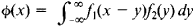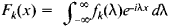# convolution

(redirected from Nonlinear convolution)
Also found in: Dictionary, Thesaurus, Medical, Legal.

## convolution

Anatomy any of the numerous convex folds or ridges of the surface of the brain

## convolution

(kon-vŏ-loo -shŏn) A mathematical operation that is performed on two functions and expresses how the shape of one is ‘smeared’ by the other. Mathematically, the convolution of the functions f(x) and g(x) is given by
(u )g(x u )du

It finds wide application in physics; it describes, for example, how the transfer function of an instrument affects the response to an input signal. See also autocorrelation function; radio-source structure.

## Convolution

The convolution of the two functions f1(x) and f2(x) is the functionThe convolution of f1(x) and f2(x) is sometimes denoted by f1 * f2

If f1 and f2 are the probability density functions of two independent random variables X and Y, then f1 * f2 is the probability density function of the random variable X + Y. If Fk(x) is the Fourier transform of the function fk(x), that is,then F1(x) F2(x) is the Fourier transform of the function f1 * f2. This property of convolutions has important applications in probability theory. The convolution of two functions exhibits an analogous property with respect to the Laplace transform; this fact underlies broad applications of convolutions in operational calculus.

The operation of convolution of functions is commutative and associative—that is, f1 * f2 = f2 * f1 and f1 * (f2 * f3) = (f1 * f2) * f3. For this reason, the convolution of two functions can be regarded as a type of multiplication. Consequently, the theory of normed rings can be applied to the study of convolutions of functions.

## convolution

[‚kän·və′lü·shən]
(anatomy)
A fold, twist, or coil of any organ, especially any one of the prominent convex parts of the brain, separated from each other by depressions or sulci.
(geology)
The process of developing convolute bedding.
A structure resulting from a convolution process, such as a small-scale but intricate fold.
(mathematics)
The convolution of the functions ƒ and g is the function F, defined by
(statistics)
A method for finding the distribution of the sum of two or more random variables; computed by direct integration or summation as contrasted with, for example, the method of characteristic functions.
Site: Follow: Share:
Open / Close